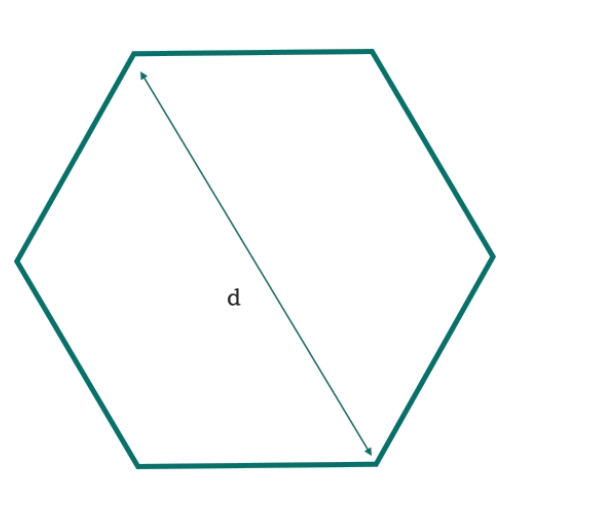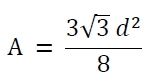# C Program for area of hexagon with given diagonal length?

Here we will see how to get the area of one hexagon using diagonal length. The diagonal length of the hexagon is d.The interior angles of a regular hexagon are 120° each. The sum of all interior angles are 720°. If the diagonal is d, then area is −## Example

#include <iostream>
#include <cmath>
using namespace std;
float area(float d) {
if (d < 0) //if d is negative it is invalid
return -1;
float area = (3 * sqrt(3) * d*d)/8.0;
return area;
}
int main() {
float r = 10;
cout << "Area : " << area(r);
}

## Output

Area : 64.9519